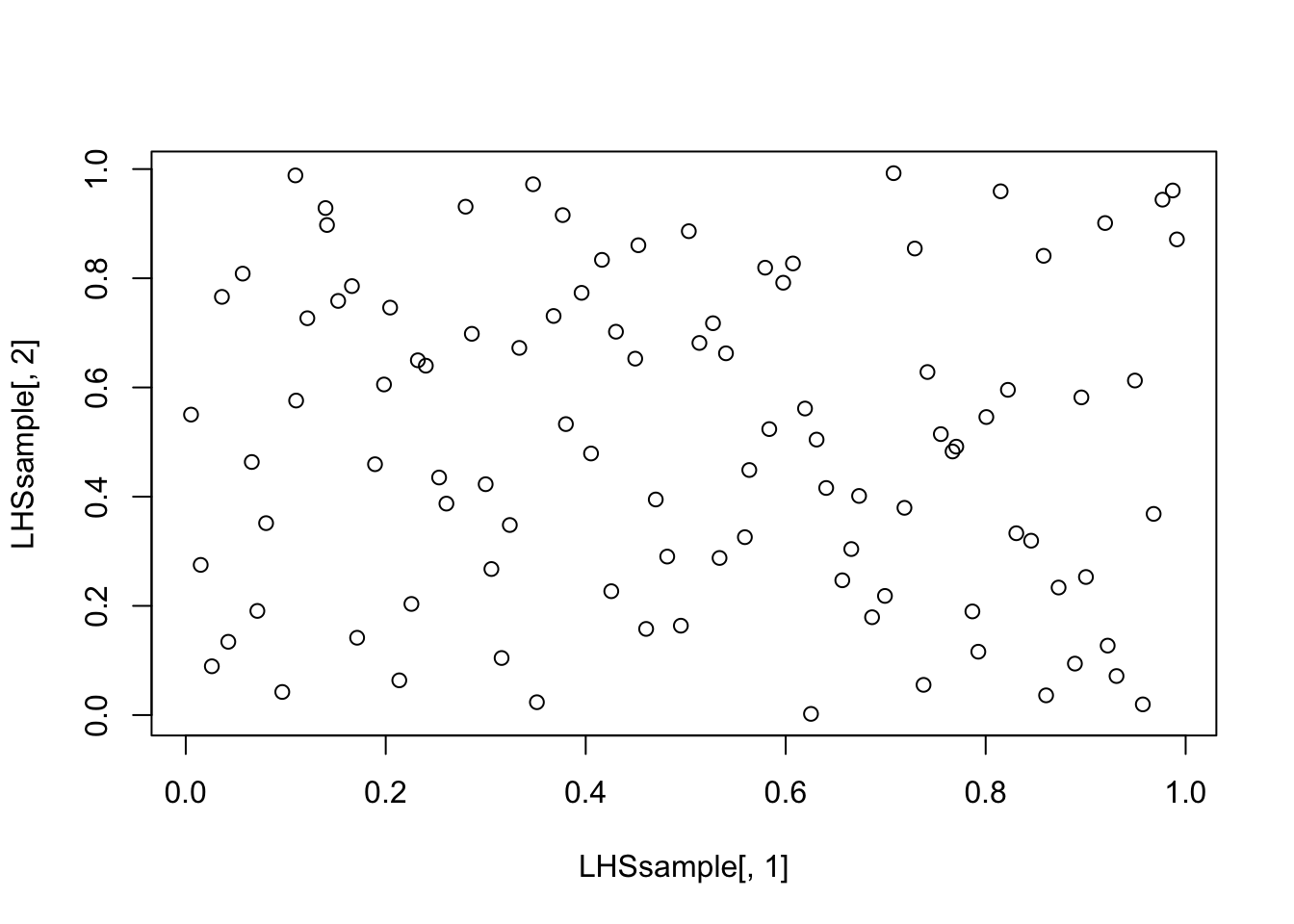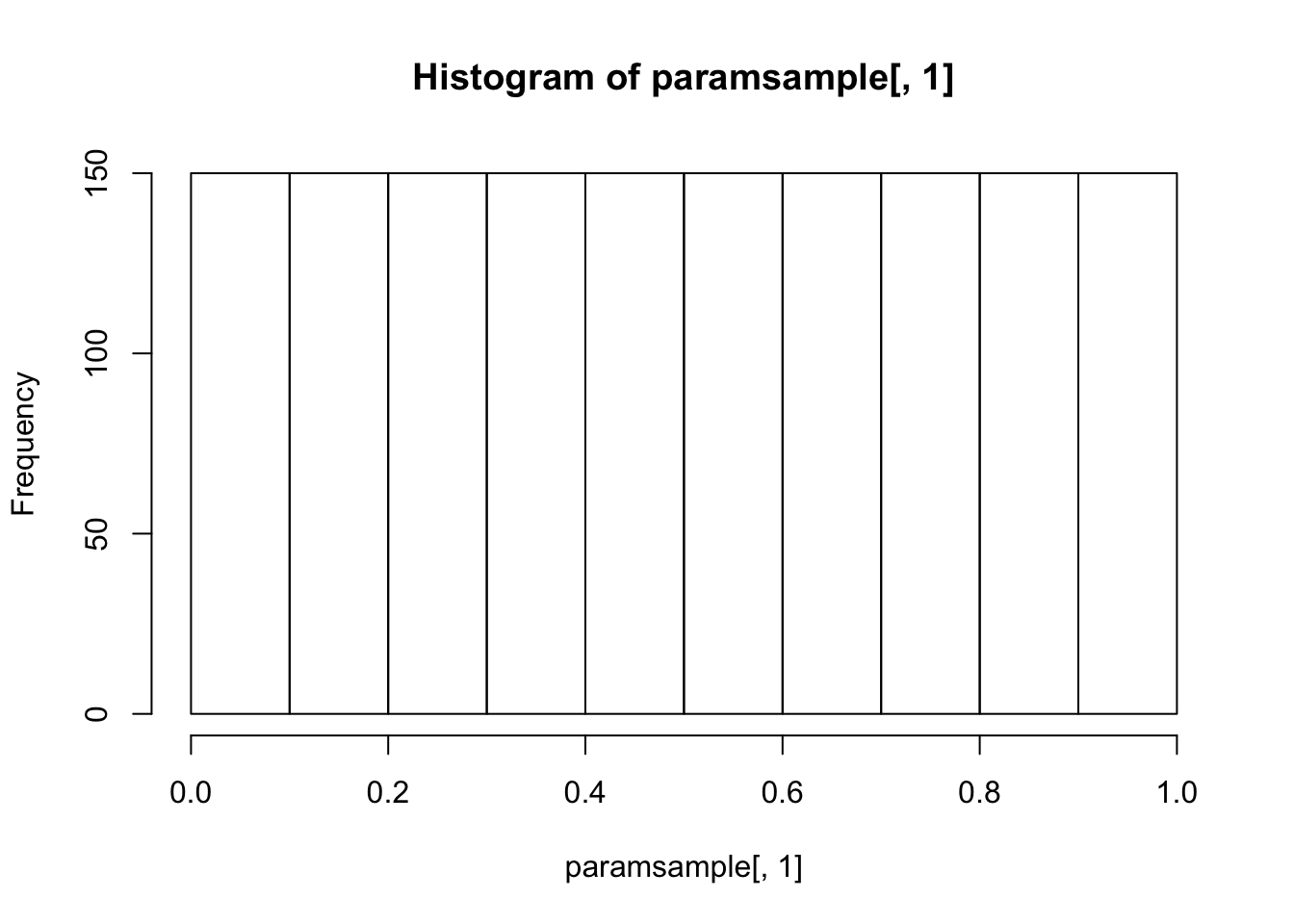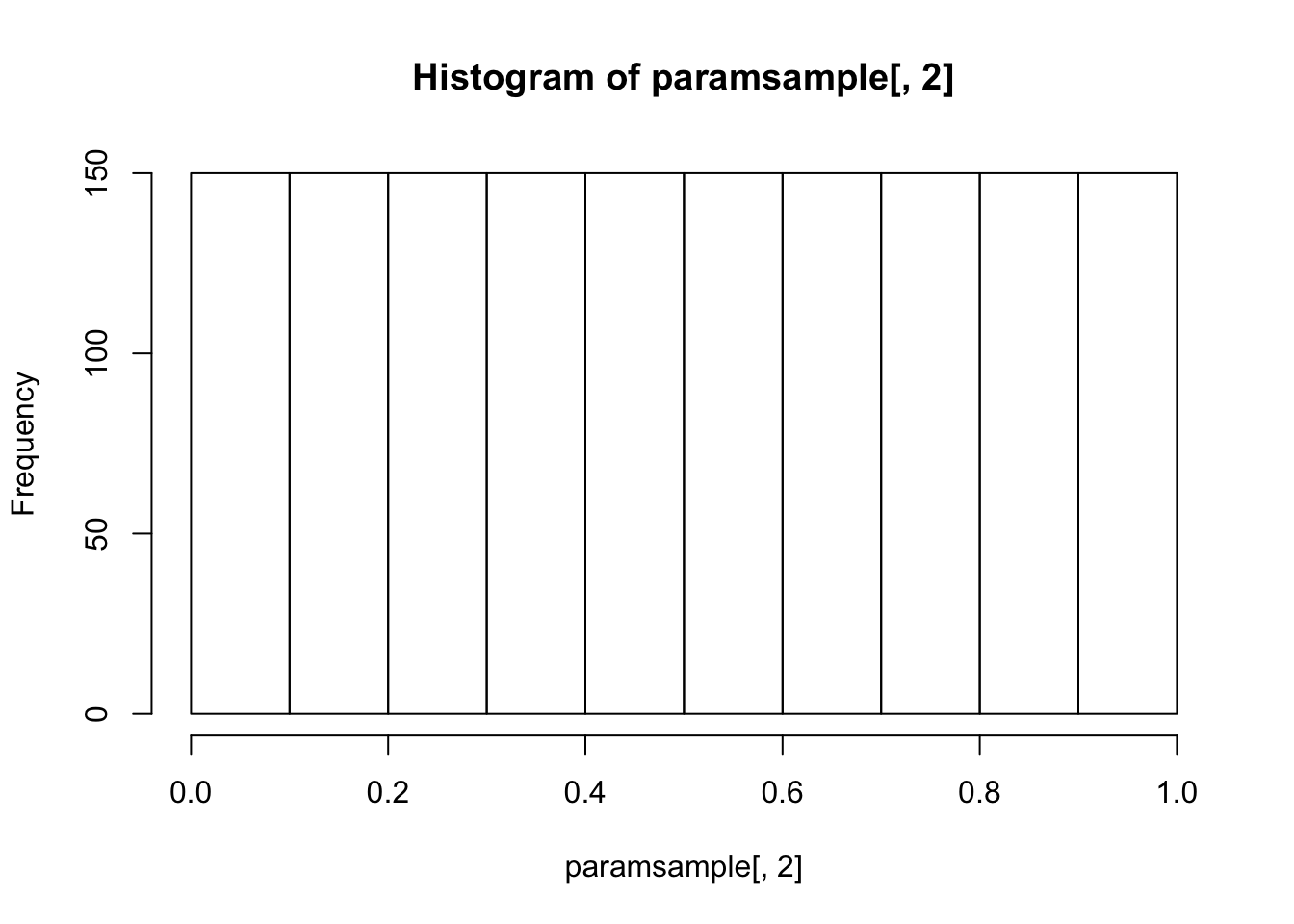We’ll use the `lhs` package to do latin hypercube sampling:

``````library(lhs)
library(deSolve)
library(ggplot2)
library(gridExtra)``````

### Latin Hypercube Sampling (LHS)

Let’s try a few different samplers and see how they look. Try the code below with several of the methods in the documentation, e.g. `randomLHS`,`geneticLHS`,`improvedLHS`, and `optimumLHS`. Do you notice any differences? Also try out the code for 1000 samples instead of 100—are any of the methods faster/slower than others?

``````set.seed(8)
LHSsample = randomLHS(100,2)
plot(LHSsample[,1],LHSsample[,2])``````### LHS for an SIR Model

Now, let’s start using LHS to look at how an SIR model behaves over a range of parameter space. We’ll start by setting up our SIR model, and including some data to work with:

``````# Data set
times = c(0, 7, 14, 21, 28, 35, 42, 49, 56, 63, 70, 77, 84, 91, 98)
data = c(97, 271, 860, 1995, 4419, 6549, 6321, 4763, 2571, 1385, 615, 302, 159, 72, 34)

# Model function
SIRode <- function(t, x, params){
S = x
I = x
R = x

b = params
g = params

dS = -b*S*I
dI = b*S*I - g*I
dR = g*I

list(c(dS, dI, dR))
}

# Extra model setup - default parameters, initial conditions, measurement equation
params = c('beta'=0.4,'gamma'=0.25, 'kappainv'=1/80000)
x0fun = function(cases,params) {
x0 = c(1-(10*params), 10*params, 0) # suppose we start with 10 cases
names(x0) = c('S0','I0','R0')
x0}
yfun = function(odeSim, params){odeSim[,3]/params} ``````

Now that we have a model, let’s generate a Latin hypercube sample for the parameters. The `lhs` package generates samples over the range (0,1), but we can rescale that to match the parameter ranges (see below).

``````numsamples = 1500
paramsample = randomLHS(numsamples,length(params))``````

First, let’s just verify that our samples do indeed appear uniform for each of the parameters:

``hist(paramsample[,1])````hist(paramsample[,2])````hist(paramsample[,3])``